# Shader入门—5.顶点动画与UV动画

``````<pre class="wp-block-syntaxhighlighter-code">Shader "Custom/VertexAnimationShader"
{
Properties
{
_Color ("Color", Color) = (1,1,1,1)
_MainTex ("Main Tex", 2D) = "white" {}

}
SubShader
{
Pass{
CGPROGRAM
#pragma vertex vert
#pragma fragment frag
#include "UnityCG.cginc"
sampler2D _MainTex;
float4 _MainTex_St;

struct a2v {
float4 vertex:POSITION;
float2 texcoord:TEXCOORD0;
};

struct v2f {
float4 pos:SV_POSITION;
float2 uv:TEXCOORD0;
};

v2f vert(a2v v){
v2f o;
o.pos=UnityViewToClipPos(v.vertex);
o.uv=v.texcoord;
return o;

}

fixed4 frag(v2f i):SV_TARGET{
return tex2D(_MainTex,i.uv);
}
ENDCG
}

}
FallBack "Diffuse"
}</pre>``````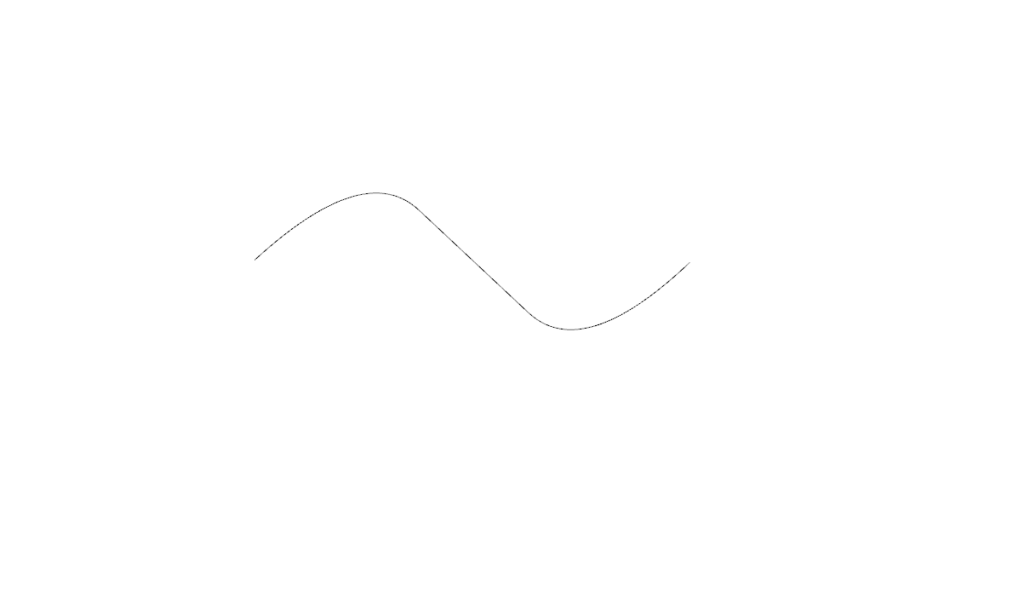二维状态下的sin函数运动规律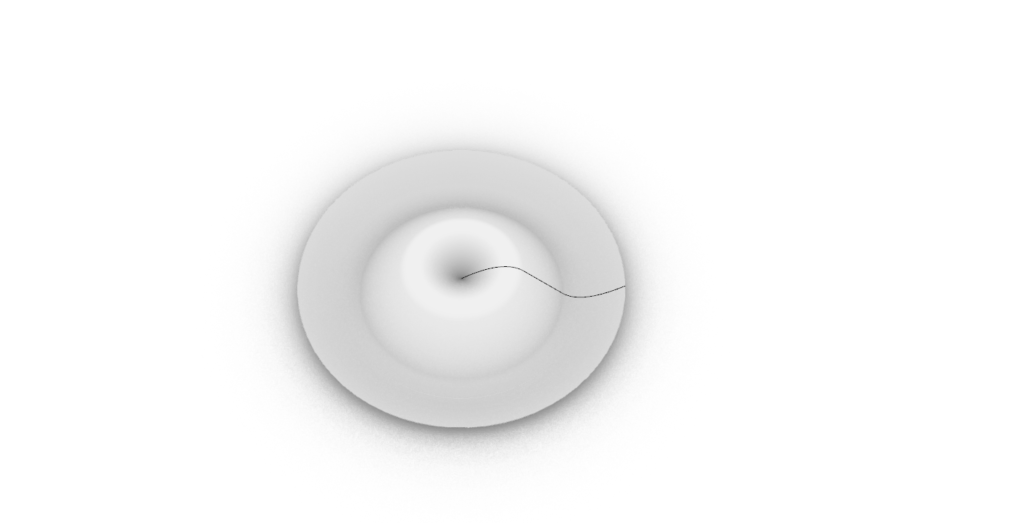在三维空间下的sin函数。

``````dis=distance(v.vertex.xyz,float3(0,0,0));
h=sin(dis*_Frequence+_Time.z)*_Magnitude``````

```Shader "Custom/VertexAnimationShader"
{
Properties
{
_Color ("Color", Color) = (1,1,1,1)
_MainTex ("Main Tex", 2D) = "white" {}
_Frequence("Frequence",Range(0,8))=2
_Magnitude("Magnitude",Range(0,8))=0.1

}
SubShader
{
Tags{"RenderType"="Qpaque"}
Pass{
CGPROGRAM
#pragma vertex vert
#pragma fragment frag
#include "UnityCG.cginc"
sampler2D _MainTex;
float4 _MainTex_ST;
float _Frequence;
float _Magnitude;

struct a2v {
float4 vertex:POSITION;
float2 texcoord:TEXCOORD0;
};

struct v2f {
float4 pos:SV_POSITION;
float2 uv:TEXCOORD0;
};

v2f vert(a2v v){
v2f o;
float dis=distance(v.vertex.xyz,float3(0,0,0));
float h=sin(dis*_Frequence+_Time.z)*_Magnitude;
o.pos=mul(unity_ObjectToWorld,v.vertex);
o.pos.y=h;
o.pos=mul(unity_WorldToObject,o.pos);
o.pos=UnityObjectToClipPos(o.pos);

o.uv = TRANSFORM_TEX(v.texcoord, _MainTex);

return o;

}

fixed4 frag(v2f i):SV_TARGET{

fixed4 col=tex2D(_MainTex,i.uv);
return col;
}
ENDCG
}

}
FallBack "Diffuse"
}

```

``float2 offset=float2(0,0);offset.x=_Time.y*_Speed;offset.y=_Time.y*_Speed;fixed4 tex=tex2D(_MovingTex,i.uv+offset);``
```Shader "Custom/VertexAnimationShader"
{
Properties
{
_Color ("Color", Color) = (1,1,1,1)
_MainTex ("Main Tex", 2D) = "white" {}
_SubTex("Sub Tex"2D)="white"{}
_Speed("Speed",Range(0,8))=2

}
SubShader
{
Tags{"RenderType"="Qpaque"}
Pass{
CGPROGRAM
#pragma vertex vert
#pragma fragment frag
#include "UnityCG.cginc"
sampler2D _MainTex;
sampler2D _SubTex;
float4 _SubTex_ST;
float4 _MainTex_ST;
float _Speed;

struct a2v {
float4 vertex:POSITION;
float2 texcoord:TEXCOORD0;
};

struct v2f {
float4 pos:SV_POSITION;
float2 uv:TEXCOORD0;
};

v2f vert(a2v v){
v2f o;
o.pos=UnityObjectToClipPos(o.pos);

o.uv = TRANSFORM_TEX(v.texcoord, _MainTex);

return o;

}

fixed4 frag(v2f i):SV_TARGET{
float2 offset=float2 (0,0);
offset.x=_Time.y*_Speed;
offset.y=_Time.y*_Speed;
fixed4 lightcol=tex2D(_SubTex，i.uv+offset)；

fixed4 col=tex2D(_MainTex,i.uv);
return col+lightcol ;
}
ENDCG
}

}
FallBack "Diffuse"
}

```

..最近才知道一个噩耗，就是unity自己的HRDP跟UE4一样无法在操作面修改管线。那我为什么不去修改UE4的管线呢。。shadergraph跟UE4的材质编辑器我依然是能做就做。但总觉得U3D吃枣药丸。唯一的优势都尼玛放弃了。

```Shader "Custom/GrassVertexAni"
{
Properties
{
_MainTex ("Texture", 2D) = "white" {}
_Noise("Noise", 2D) = "black" {}
_WindControl("WindControl(x:XSpeed y:YSpeed z:ZSpeed w:windMagnitude)",vector) = (1,0,1,0.5)
//前面几个分量表示在各个轴向上自身摆动的速度, w表示摆动的强度
_WaveControl("WaveControl(x:XSpeed y:YSpeed z:ZSpeed w:worldSize)",vector) = (1,0,1,1)
//前面几个分量表示在各个轴向上风浪的速度, w用来模拟地图的大小,值越小草摆动的越凌乱，越大摆动的越整体
}
SubShader
{
Tags { "Queue" = "Transparent"}

Pass
{
//Tags{"LightMode"="ForwardBase"}
//ZWrite Off
Blend SrcAlpha OneMinusSrcAlpha
CGPROGRAM

#pragma vertex vert
#pragma fragment frag
#pragma multi_compile_instancing

#include "UnityCG.cginc"

struct appdata
{
float4 vertex : POSITION;
float2 uv : TEXCOORD0;
UNITY_VERTEX_INPUT_INSTANCE_ID
};

struct v2f
{
float2 uv : TEXCOORD0;
float4 pos : SV_POSITION;
//float3 tempCol : TEXCOORD1;//用来测试传递noise贴图采样的结果
};

sampler2D _MainTex;
sampler2D _Noise;
half4 _WindControl;
half4 _WaveControl;

v2f vert (appdata v)
{
v2f o;
UNITY_SETUP_INSTANCE_ID(v);
float4 worldPos = mul(unity_ObjectToWorld, v.vertex);
float2 samplePos = worldPos.xz / _WaveControl.w;
samplePos += _Time.x * -_WaveControl.xz;
fixed waveSample = tex2Dlod(_Noise, float4(samplePos, 0, 0)).r;
worldPos.x += sin(waveSample * _WindControl.x) * _WaveControl.x * _WindControl.w * v.uv.y;
worldPos.z += sin(waveSample * _WindControl.z) * _WaveControl.z * _WindControl.w * v.uv.y;
o.pos = mul(UNITY_MATRIX_VP, worldPos);
o.uv = v.uv;
//o.tempCol = waveSample;

return o;
}

fixed4 frag (v2f i) : SV_Target
{
fixed4 col = tex2D(_MainTex, i.uv);
//return fixed4(frac(i.tempCol.x), 0, 0, 1);
return col;
}
ENDCG
}
}
}
```

# Shader入门—4.卡通材质（2.正式制作Toon-Shading）左手坐标系

Z轴代表我们的深度坐标，越远z的值越大，而我们的卡通材质需要我们清晰的看到他的描边防止被正面渲染的部分给遮挡住所以进行统一的-0.5的定值。所以我们正式来编写描边的Pass。

```Shader"Custom/Toon-Shading"{
Properties{
_Outline("Outline Size",Range(0,1))=0.5
_OutLineColor("OutLine Color",Color)=(1,1,1,1)
_Color("Color Tint",Color)=(1,1,1,1)
}
Subshader{
Pass{
NAME "OUTLINE"
Cull Front
CGPROGRAM

#pragma vertex vert
#pragma fragmengt frag
#include "Lighting.cginc"
#include "UnityCG.cginc"
struct a2v{
float4 vertex:POSITION;
float3 normal:NORMAL;
};
struct v2f{
float4 pos:SV_POSITION;
};
v2f vert(a2v v){
v2f o;
float4 pos=UnityObjectToClipPos(v.vertex);
float3 normal=mul((float3x3)UNITY_MATRIX_IT_MV,v.normal);
normal.z=-0.5;
pos=pos+float4(normalize(normal),0)*_Outline;
o.pos=mul(UNITY_MATRIX_P,pos);
return o;
}
fixed4 frag(v2f i):SV_Target{
return float4(_OutLineColor.rgb,1);
}
ENDCG
}
}
Fallback "Diffuse"
}
```

``Specular=Clight*Mdiffuse*max(0,n·halfDir)^_gloss``

``fixed4 specular=_LightColor0.rgb*_Diffuse*pow(max(0,dot(normal,halfDir)),_Gloss);``

``float spec=dot(worldNormal,halfDir);spec=step(a,spec);``

``Shader "Custom/Toon-ShadingShader2"{    Properties    {        _Color ("Color", Color) = (1,1,1,1)        _Specular("Specular Color",Color)=(1,1,1,1)        _SpecularScale("Specular Scale",Range(0,0.1))=0.1        _Outline("Outline Size",Range(0,1))=0.5        _OutlineColor("Outline Color",Color)=(1,1,1,1)        _MainTex("Main Tex",2D)="white"{}    }    SubShader    {        Tags{"RenderType"="Opaque"  "Queue"="Geometry"}                Pass{                                    NAME "OUTLINE"            Cull Front            CGPROGRAM            #pragma vertex vert            #pragma fragment frag             #include "Lighting.cginc"            #include "UnityCG.cginc"            float _Outline;            fixed4 _OutlineColor;            struct a2v{               float4 vertex:POSITION;               float3 normal:NORMAL;            };            struct v2f{                float4 pos:SV_POSITION;                            };            v2f vert(a2v v){                v2f o;                float4 pos=UnityObjectToClipPos(v.vertex);                float3 normal=mul((float3x3)UNITY_MATRIX_IT_MV,v.normal);                normal.z=-0.5;                pos=pos+float4(normalize(normal),0)*_Outline;                o.pos=mul(UNITY_MATRIX_P,pos);                return o;                            }            fixed4 frag(v2f i):SV_TARGET{                return float4(_OutlineColor.rgb,1.0);            }                        ENDCG        }        Pass{            Tags{"LightMode"="ForwardBase"}            Cull Back            CGPROGRAM                ...            ENDCG        }    }    FallBack "Diffuse"}``

``DIRECTIONAL LIGHTMAP_ON DIRLIGHTMAP_ON SHADOWS_OFF``

``                        #pragma vertex vert             #pragma fragment frag             #pragma multi_compile_fwdbase            #include "UnityCg.cginc"            #include "Lighting.cginc"            #include "AutoLight.cginc"            #include "UnityShaderVariables.cginc"``

``            fixed4 _Color;            sampler2D _MainTex;            float4 _MainTex_ST;            fixed4 _Specular;            fixed _SpecularScale;``

``            struct a2v{                float4 vertex:POSITION;                float3 normal:NORMAL;                float4 texcoord:TEXCOORD0;                            };``

``            v2f vert(a2v v){                v2f o;                o.pos=UnityObjectToClipPos(v.vertex);                o.uv=TRANSFORM_TEX(v.texcoord,_MainTex);                o.worldNormal=UnityObjectToWorldNormal(v.normal);                o.worldPos=mul(unity_ObjectToWorld,v.vertex).xyz;                TRANSFER_SHADOW(o);                return o;            }``

``            struct v2f {                float4 pos:SV_POSITION;                float2 uv:TEXCOORD0;                float3 worldNormal:TEXCOORD1;                float3 worldPos:TEXCOORD2;                SHADOW_COORDS(3)            };``

``            fixed4 frag(v2f i):SV_TARGET{                float3 worldNormal=normalize(i.worldNormal);                float3 worldLightDir=normalize(UnityWorldSpaceLightDir(i.worldPos));                float3 worldViewDir=normalize(UnityWorldSpaceViewDir(i.worldPos));                float3 halfDir=normalize(worldLightDir+worldViewDir);                            }``

``fixed3 tex=tex2d(_MainTex,i.uv);fixed3 albedo=tex.rgb*_Color;``

``fixed3 ambient=UNITY_LIGHTMODEL_AMBIENT.xyz*albedo;``

``saturate(0,dot(worldNormal,worldLightDir));``

``diff=dot(worldNormal,worldLightDir);diff=0.5*diff+0.5;``

``UNITY_LIGHT_ATTENUATION(atten,i,i.worldPos);``

``diff=(0.5*diff+0.5)*atten;``

``Shader"Custom/Toon-Shading"{    Properties{        ...        _Ramp("Ramp Texture",2D)="white"{}        ...    }    Subshader{        ...        sampler2D _Ramp;        float _Ramp_ST;        ...       fixed4 diffuse=_LightColor0.rgb*albedo*tex2D(_Ramp,float2(diff,diff)).rgb;``

specular=Clight*Mdiffuse*max(0,n*halfDir)^Gloss 然后我们改进后的一部分公式smoothstep(-w,w,spec-a)，其中w是我们通过fwidth函数实现的，而fwidth这个函数在CG语言中的含义如下：`fwidth — return the sum of the absolute value of derivatives in x and y` 意思是返回x，y中导数绝对值的和，但我们只需要临近像素之间的近似导数值，而高光部分我们只需要如此计算：fixed w=fwidth(dot(worldNormal,halfDir))*2则可

``fixed w=fwidth(dot(worldNormal.halfDir))*2.0;fixed3 specular=_Specular.rgb*lerp(0,1,smoothstep(-w,w,spec+_SpecularScale-1));``

``fixed3 specular=_Specular.rgb*lerp(0,1,smoothstep(-w,w,spec+_SpecualrScale-1))*step(0.00001,_SpecularScale);``

```Shader "Custom/Toon-ShadingShader2"
{
Properties
{
_Color ("Color", Color) = (1,1,1,1)
_Specular("Specular Color",Color)=(1,1,1,1)
_SpecularScale("Specular Scale",Range(0,0.1))=0.1
_Outline("Outline Size",Range(0,1))=0.5
_OutlineColor("Outline Color",Color)=(1,1,1,1)
_MainTex("Main Tex",2D)="white"{}
_Ramp("Ramp Texture",2D)="white"{}
}
SubShader
{
Tags{"RenderType"="Opaque"  "Queue"="Geometry"}

Pass{

NAME "OUTLINE"
Cull Front
CGPROGRAM
#pragma vertex vert
#pragma fragment frag
#include "Lighting.cginc"
#include "UnityCG.cginc"
float _Outline;
fixed4 _OutlineColor;
struct a2v{
float4 vertex:POSITION;
float3 normal:NORMAL;
};
struct v2f{
float4 pos:SV_POSITION;

};
v2f vert(a2v v){
v2f o;
float4 pos=UnityObjectToClipPos(v.vertex);
float3 normal=mul((float3x3)UNITY_MATRIX_IT_MV,v.normal);
normal.z=-0.5;
pos=pos+float4(normalize(normal),0)*_Outline;
o.pos=mul(UNITY_MATRIX_P,pos);
return o;

}
fixed4 frag(v2f i):SV_TARGET{
return float4(_OutlineColor.rgb,1.0);
}

ENDCG
}
Pass{
Tags{"LightMode"="ForwardBase"}
Cull Back
CGPROGRAM
#pragma vertex vert
#pragma fragment frag
#pragma multi_compile_fwdbase
#include "UnityCg.cginc"
#include "Lighting.cginc"
#include "AutoLight.cginc"
#include "UnityShaderVariables.cginc"
fixed4 _Color;
sampler2D _MainTex;
float4 _MainTex_ST;
fixed4 _Specular;
fixed _SpecularScale;
sampler2D _Ramp;
float _Ramp_ST;

struct a2v{
float4 vertex:POSITION;
float3 normal:NORMAL;
float4 texcoord:TEXCOORD0;

};
struct v2f {
float4 pos:SV_POSITION;
float2 uv:TEXCOORD0;
float3 worldNormal:TEXCOORD1;
float3 worldPos:TEXCOORD2;
SHADOW_COORDS(3)
};

v2f vert(a2v v){
v2f o;
o.pos=UnityObjectToClipPos(v.vertex);
o.uv=TRANSFORM_TEX(v.texcoord,_MainTex);
o.worldNormal=UnityObjectToWorldNormal(v.normal);
o.worldPos=mul(unity_ObjectToWorld,v.vertex).xyz;
TRANSFER_SHADOW(o);
return o;
}

fixed4 frag(v2f i):SV_TARGET{
fixed3 worldNormal=normalize(i.worldNormal);
fixed3 worldLightDir=normalize(UnityWorldSpaceLightDir(i.worldPos));
fixed3 worldViewDir=normalize(UnityWorldSpaceViewDir(i.worldPos));
fixed3 halfDir=normalize(worldLightDir+worldViewDir);

fixed3 tex=tex2D(_MainTex,i.uv);
fixed3 albedo=tex.rgb*_Color.rgb;
fixed3 ambient=UNITY_LIGHTMODEL_AMBIENT.xyz*albedo;
fixed diff=dot(worldNormal,worldLightDir);
UNITY_LIGHT_ATTENUATION(atten,i,i.worldPos);
diff=(diff*0.5+0.5)*atten;
fixed3 diffuse=_LightColor0.rgb*albedo*tex2D(_Ramp,float2(diff,diff)).rgb;
fixed w=fwidth(dot(worldNormal,halfDir))*2.0;
fixed3 specular=_Specular*lerp(0,1,smoothstep(-w,w,dot(worldNormal,halfDir)+_SpecularScale-1))*step(0.0001,_SpecularScale);
return fixed4 (ambient+diffuse+specular,1.0);
}
ENDCG
}
}
FallBack "Diffuse"
}

```

```void LWRPLightingFunction_float (float3 ObjPos, out float3 Direction, out float3 Color, out float ShadowAttenuation )
{
#ifdef LIGHTWEIGHT_LIGHTING_INCLUDED
#define LIGHTWEIGHT_LIGHT_INCLUDE
//Actual light data from the pipeline
Light light = GetMainLight(GetShadowCoord(GetVertexPositionInputs(ObjPos)));
Direction = light.direction;
Color = light.color;
ShadowAttenuation = light.shadowAttenuation;

#else

//Hardcoded data, used for the preview shader inside the graph
//where light functions are not available
Direction = float3(-0.5, 0.5, -0.5);
Color = float3(1, 1, 1);
ShadowAttenuation = 0.4;

#endif
}
```

# Shader入门—4.卡通材质（1.Blinn-Phong模型）

1.Blinn-Phong高光模型结合漫反射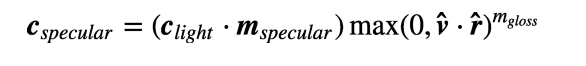Phong高光模型phong模型计算高光高光反射的计算公式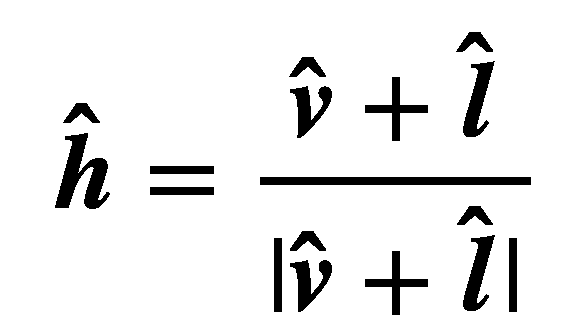h的计算过程

``fixed3 reflectDir=normalize(reflect(-worldLightDir,worldNormal));fixed3 viewDir=normalize(_WorldSpaceCammeraPos.xyz-unity_ObjectToWorld(v.vertex).xyz);``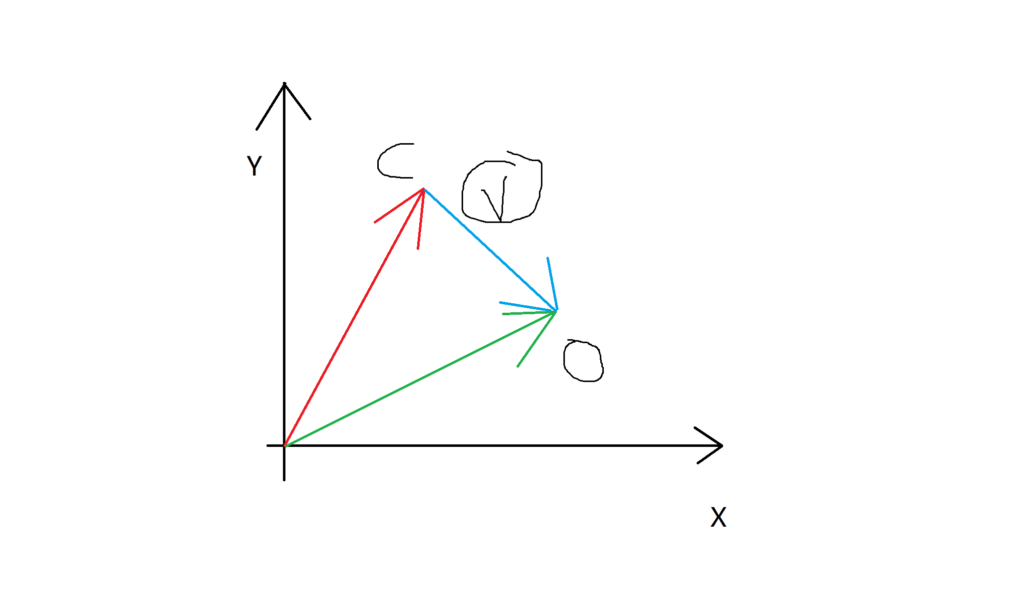viewDir的具象化

``fixed3 specular=_LightColor0.rgb*_Specular.rgb*pow(saturate(dot(viewDir,reflectDir)),_Gloss)；``

``Shader "Custom/DiffuseShader"{    Properties    {        _Diffuse("Diffuse Color",Color)=(1,1,1,1)        _Specular("Specular Color",Color)=(1,1,1,1)        _Gloss("Gloss",Range(8,255))=20    }    SubShader    {        pass{            Tags{"LightModel"="ForwardBase"}            CGPROGRAM                        #pragma vertex vert            #pragma fragment frag            #include "Lighting.cginc"            fixed4 _Diffuse;            fixed4 _Specular;            float _Gloss;            struct a2v{                float4 vertex : POSITION;                float3 normal : NORMAL;            };            struct v2f{                float4 pos:SV_POSITION;                float3 color:COLOR;            };            v2f vert(a2v v){                v2f o;                o.pos=UnityObjectToClipPos(v.vertex);                fixed3 ambient=UNITY_LIGHTMODEL_AMBIENT.xyz;                fixed3 worldNormal=normalize(mul(v.normal,(float3x3)unity_WorldToObject));                                fixed3 worldLightDir=normalize(_WorldSpaceLightPos0.xyz);                fixed3 lightNormal=normalize(_WorldSpaceLightPos0);                fixed3 diffuse=_LightColor0.rgb*_Diffuse.rgb*saturate(dot(worldNormal,lightNormal));                fixed3 reflectDir=normalize(reflect(-WorldLightDir,worldNormal));                fixed3 viewDir=normalize(_WorldSpaceCameraPos.xyz-unity_ObjectToWorld(v.vertex).xyz);                fixed3 specular=_LightColor0.rgb*_Specular*pow(saturate(dot(viewDir,reflectDir)),_Gloss);                                                o.color=ambient.rgb+diffuse.rgb+specular;                return o;            }            fixed4 frag(v2f i):SV_TARGET{                return fixed4(i.color,1.0);            }            ENDCG        }    }    FallBack "Diffuse"}``

n是物体表面法线向量，h是新定义的光源向量与视觉方向取平均后的归一化。在代码中我们可以直接将worldLightDir与viewDir相加然后进行归一化计算就行了。所以这Blinn-Phong的高光部分如下代码呈现

``fixed3 viewDir=normalize(_WorldSpaceCameraPos.xyz-unity_ObjectToWorld(v.normal).xyz);fixed3 halfDir=normalize(worldLightDir,viewDir);``

``fixed3 diffuse=_LightColor0.rgb*_Specular*pow(max(0,dot(worldNormal,halfDir)),_Gloss);``

``Shader "Custom/Blinn-PhongSpecularDiffuseShader"{    Properties{        _Diffuse("Diffuse Color",Color)=(1,1,1,1)        _Specular("Specular Color",Color)=(1,1,1,1)        _Gloss("Gloss",Range(8,255))=20    }    SubShader{        Pass{            Tags{"LightMode"="ForwardBase"}            CGPROGRAM            #pragma vertex vert            #pragma fragment frag            #include "Lighting.cginc"            fixed4 _Diffuse;            fixed4 _Specular;            float  _Gloss;            struct a2v{                float4 vertex:POSITION;                float3 normal:NORMAL;            };            struct v2f{                float4 pos:SV_POSITION;                fixed3 color:COLOR;            };            v2f vert(a2v v){                v2f o;                o.pos=UnityObjectToClipPos(v.vertex);                fixed3 worldNormal=normalize(mul(v.normal,(float3x3)unity_WorldToObject));                fixed3 lightDir=normalize(_WorldSpaceLightPos0.xyz);                fixed3 diffuse=_LightColor0.rgb*_Diffuse*saturate(dot(worldNormal,lightDir));                fixed3 ambient=UNITY_LIGHTMODEL_AMBIENT.xyz;                fixed3 viewDir=normalize(_WorldSpaceCameraPos.xyz-UnityObjectToWorld(v.normal).xyz);                fixed3 halfDir=normalize(lightDir+viewDir);                fixed3 specular=_LightColor0.rgb*_Specular*pow(max(0,dot(worldNormal,halfDir)),_Gloss);                o.color=ambient+diffuse+specular;                return o;            }            fixed4 frag(v2f i):SV_TARGET{                return fixed4(i.color,1.0);            }            ENDCG        }    }    FallBack "Diffuse"}``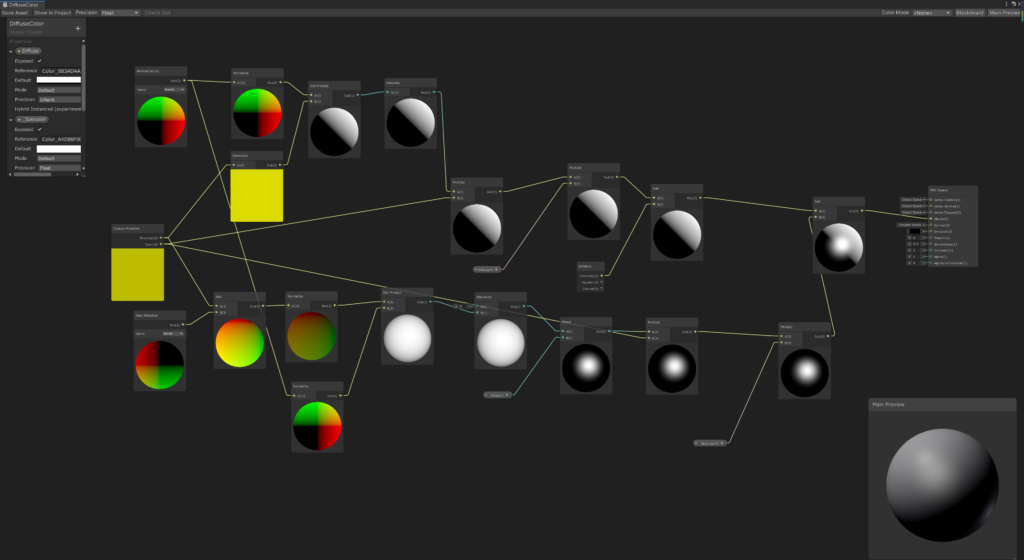Blinn-Phong高光漫反射模型

# Shader入门—3.漫反射光照模型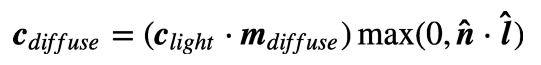兰伯特定律

n是表面法线，l是光源的单位矢量，max（0，n·l）是取0，n·l中的最大值，clight 是光源颜色，mdiffuse 是材质的漫反射颜色。

``Shader "Custom/DiffuseShader"{    Properties    {        _Diffuse("Diffuse Color",Color)=(1,1,1,1)    }    SubShader    {        pass{            Tags{"LightModel"="ForwardBase"}            CGPROGRAM                        #pragma vertex vert            #pragma fragment frag            #include "Lighting.cginc"            fixed4 _Diffuse;            struct a2v{                float4 vertex : POSITION;                float3 normal : NORMAL;            };            struct v2f{                float4 pos:SV_POSITION;                float3 color:COLOR;            };            v2f vert(a2v v){                v2f o;                o.pos=UnityObjectToClipPos(v.vertex);                fixed3 ambient=UNITY_LIGHTMODEL_AMBIENT.xyz;                fixed3 worldNormal=normalize(mul(v.normal,(float3x3)unity_WorldToObject));                fixed3 lightNormal=normalize(_WorldSpaceLightPos0);                fixed3 diffuse=_LightColor0.rgb*_Diffuse.rgb*saturate(dot(worldNormal,lightNormal));                o.color=ambient.rgb+diffuse.rgb;                return o;            }            fixed4 frag(v2f i):SV_TARGET{                return fixed4(i.color,1.0);            }            ENDCG        }    }    FallBack "Diffuse"}``

``fixed4 _Diffuse;``

_Diffuse是颜色属性，我们按照惯例直接定义成fixed4类型。

``Struct a2v{    float4 vertex:POSITION;    float3 normal:NORMAL;};``

``v2f vert(a2v v){    v2f o;    o.pos=UnityObjectToClipPos(v.vertex);    ...}``

v2f 定义了我们的输出参数是o，之后的就是一般设置。顶点的法线信息是基于模型坐标的，所以我们首先将其变换到空间坐标中，这里需要将顶点的法线与变换矩阵unity_WorldToObject（老版本为：_World2Object）进行mul计算,之后将其归一化，所以我们这么写

``fixed3 worldNormal=normalize(mul(v.normal,unity_WorldToObject));``

``fixed3 worldNormal=normalize(mul(v.normal,(float3x3)unity_WorldToObject));``

``fixed3 worldLight=normalize(_WorldSpaceLightPos0);``

``fixed3 diffuse=_LightColor0.rgb*_Diffuse.rgb*saturate(worldNormal,worldLight);``

``fixed3 ambient=UNITY_LIGHTMODEL_AMBIENT.xyz;``

``            v2f vert(a2v v){                v2f o;                o.pos=UnityObjectToClipPos(v.vertex);                fixed3 ambient=UNITY_LIGHTMODEL_AMBIENT.xyz;                fixed3 worldNormal=normalize(mul(v.normal,(float3x3)unity_WorldToObject));                fixed3 lightNormal=normalize(_WorldSpaceLightPos0);                fixed3 diffuse=_LightColor0.rgb*_Diffuse.rgb*saturate(dot(worldNormal,lightNormal));                o.color=ambient.rgb+diffuse.rgb;                return o;            }``

``struct v2f{    pos:SV_POSITION;    color:COLOR;};``

``fixed4 frag(v2f i):SV_Target{    return fixed4 (i.color,1.0)}``shader graph中的Lambert漫反射模型自定义节点

``#if SHADERGRAPH_PREVIEW        Direction=half3(0.5,0.5,0); Color=1;#else        Light light=GetMainlight();         Direction=light.direction;         Color=light.color;#endif``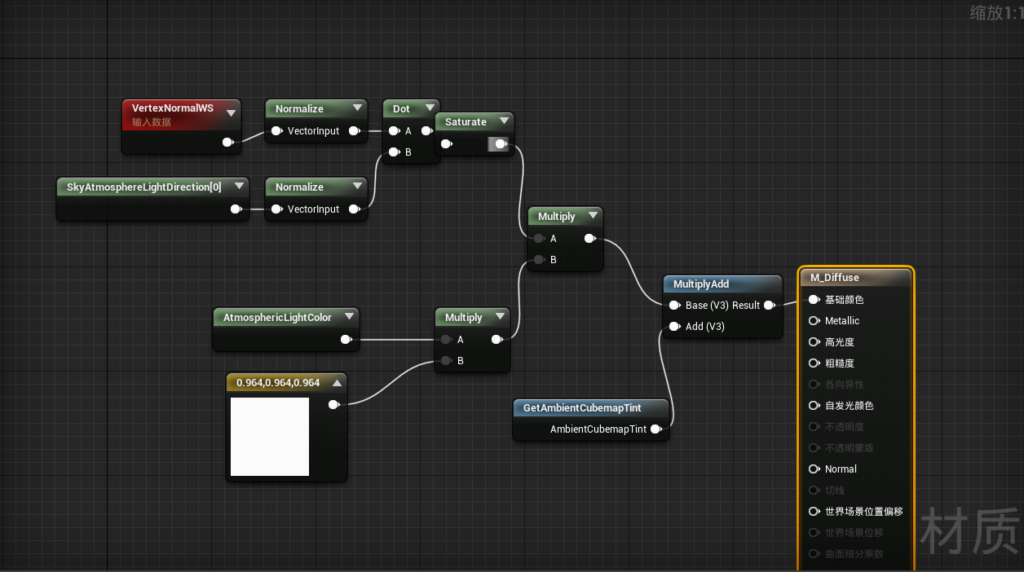UE4中的shader

# Shader入门—EX:BRDF光照模型(在施工）

BRDF有两种理解，第一种是当给定入射角度的时候，BRDF可以给出所有出射方向上的反射和散射光线的相对分布情况。注意，BRDF模型并不是真实的光照模型，而是看起来对的光照模型，这是一个经验模型。第二种理解是，当给定观察方向的时候，BRDF可以给出所有入射方向到出射方向的光线分布。

Filament中基于物理的渲染

# Shader入门——2贴图材质

``````Shader"Custom/BaseTextureMatShader"
{
Properties{
_Color("Color Tint",Color)=(1.0,1.0,1.0,1.0)
_MainTex("Main Texture",2D)="white"
}
Subshader{
Pass{
CGPROGRAM
#pragma vertex vert
#pragma fragmengt frag
fixed4 _Color;
sampler2D _MainTex;

float4 vert(float4 pos:POSITION):SV_POSITION{
return UnityObjectToClipPos(pos);
}

fixed4 frag(float2 uv:TEXCOORD0):SV_Target{
return tex2D(_MainTex,uv);
}

ENDCG
}
}
Fallback "Diffuse"
}
``````

``````sampler2D _MainTex;
fixed4 _Color;``````

``````#pragma vertex vert
#pragma fragmengt frag``````

``````float4 vert(float4 vertex:POSITION):SV_POSITION{
return UnityObjectToClipPos(vertex);
}``````

``````fixed4 frag( float2 uv:TEXCOORD0):SV_Target{
return tex2D(_MainTex,uv);
}``````

``````Shader"Custom/textureShader"{
Properties{
_MainTex("Main Tex",2D)="white"{}
_Color("COlor Tint",Color)=(1,1,1,1)
}
Subshader{
Pass{
CGPROGRAM
#pragma vertex vert
#pragma fragment frag

fixed4 _Color;
sampler2D _MainTex;

float4 vert (float4 vertex:POSITION):SV_POSITION{
return UnityObjectToClipPos(vertex);
}

fixed4 frag(float2 uv:TEXCOORD0):SV_Target{
return tex2D(_MainTex,uv);
}

ENDCG
}

}
Fallback "Diffuse"
}``````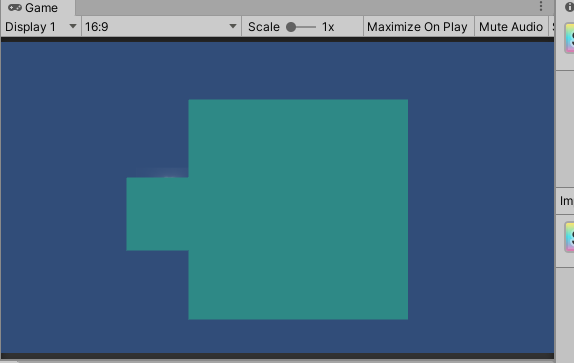我们并不是想要这种纯色的贴图，而是更加丰富的

``````struct a2v{
float4 vertex:POSITION;
float2 uv:TEXCOORD0;
};``````

``````v2f vert(a2v v):SV_POSITION{
v2f o;
o.vertex=UnityObjectToClipPos(v.vertex);
o.uv=v.uv;
return o;
}``````

``````struct v2f{
float4 pos:SV_POSITION;
float2 uv:TEXCOORD1;
};``````

``````fixed4 frag(v2f i):SV_Target{
return tex2D(_MainTex,i.uv)
}``````

``````Shader"Custom/textureShader"{
Properties{
_MainTex("Main Tex",2D) = "white"{}
_Color("COlor Tint",Color) = (1,1,1,1)
}
Subshader{
Pass{
CGPROGRAM
#pragma vertex vert
#pragma fragment frag

fixed4 _Color;
sampler2D _MainTex;

struct a2v {
float4 vertex:POSITION;
float4 uv:TEXCOORD0;
};

struct v2f {
float4 pos:SV_POSITION;
float2 uv:TEXCOORD1;
};

v2f vert(a2v v):SV_POSITION {
v2f o;
o.pos = UnityObjectToClipPos(v.vertex);
o.uv = v.uv;
return o;
}

fixed4 frag(v2f i) :SV_Target{
return tex2D(_MainTex,i.uv);
}

ENDCG
}

}
Fallback "Diffuse"
}``````

``invalid output semantic 'SV_POSITION': Legal indices are in [0,0]``

``````Shader"Custom/textureShader"{
Properties{
_MainTex("Main Tex",2D) = "white"{}
_Color("COlor Tint",Color) = (1,1,1,1)
}
Subshader{
Pass{
CGPROGRAM
#pragma vertex vert
#pragma fragment frag

fixed4 _Color;
sampler2D _MainTex;

struct a2v {
float4 vertex:POSITION;
float4 uv:TEXCOORD0;
};

struct v2f {
float4 pos:SV_POSITION;
float2 uv:TEXCOORD1;
};

v2f vert(a2v v){
v2f o;
o.pos = UnityObjectToClipPos(v.vertex);
o.uv = v.uv;
return o;
}

fixed4 frag(v2f i) :SV_Target{
return tex2D(_MainTex,i.uv);
}

ENDCG
}

}
Fallback "Diffuse"
}``````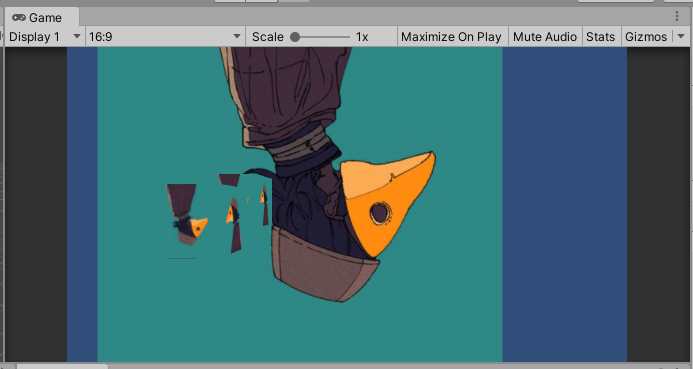正确输出的贴图纹理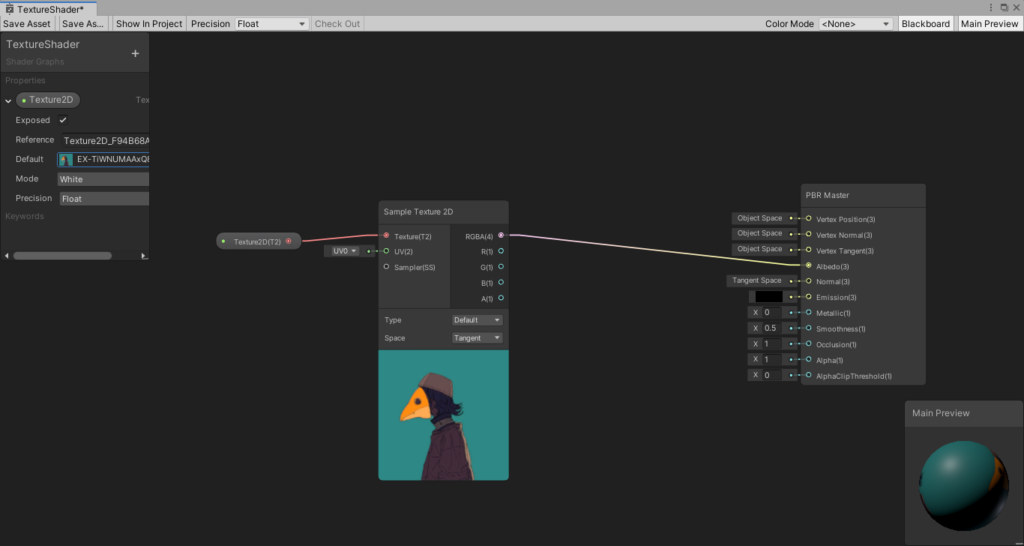shader graph中的2D纹理贴图UE4中的纹理贴图

SD中进行纹理贴图与UE4中相似，但贴图节点的名称为bitmap。SD中的bitmap节点

bitmap主要存储的是一个rgba的像素组，但SD作为一个造轮子的软件，他的材质不需要对geometry负责，所以一般不会在SD中进行使用贴图，也有其他的情况需要进行赋予贴图，这里不再赘述。

# Shader入门——1.基本框架

``````Shader "Custom/RgbShader"
{
Properties
{
_Color ("Color", Color) = (1,1,1,1)
_MainTex ("Albedo (RGB)", 2D) = "white" {}
_Glossiness ("Smoothness", Range(0,1)) = 0.5
_Metallic ("Metallic", Range(0,1)) = 0.0
}
SubShader
{
Pass
{
CGPROGRAM

#pragma vertex vert
float4 vert(float4 pos:POSITION):POSITION
{
return UnityObjectToClipPos(pos);
}

#pragma fragment frag
fixed4 frag():COLOR
{
return fixed4(1,0,0,1);
}
ENDCG
}
}
FallBack "Diffuse"
}``````

``````Shader"Custom/ShaderName"
Properties{
_Color{"Color",Color}=(1,1,1,1)
...
}``````

``````Shader"Custom/ShaderName"
Properties{
_Color("Color",Color)=(1,1,1,1)
}
Subshader{
Pass{
}
}
Fallback "Diffuse"``````

``````Shader"Custom/ShaderName"
Properties{
_Color("Color",Color)=(1,1,1,1)
...
}
Subshader{
Pass{
CGPROGRAM
...
ENDCG
}
}
Fallback "Diffuse"``````

``#pragma vertex vert``

``````Shader"Custom/ShaderName"
Properties{
_Color("Color",Color)=(1,1,1,1)
...
}
Subshader{
Pass{
CGPROGRAM
#pragma vertex vert
#pragma fragment frag
...
ENDCG
}
}
Fallback "Diffuse"``````

``````float4 vert(float4 pos:POSITION):SV_PSOITION{
}``````

``return UnityObjectToClipPos(pos);``

UnityObjectToClipPos（）是新版unityshader的内置函数，作用就是相当于原来之前的mul(UNITY_MATRIX_MVP,pos);矩阵变换 。该矩阵变换是将当前的模型的顶点/方向矢量从模型空间变换到裁剪空间。所以完整的顶点着色器的代码如下。（mul是multiple的缩写，在这指乘法）

``````float4 vert(pos:POSITION):SV_POSITION{
return UnityObjectToClipPos(pos);
}``````

``````fixed4 frag():SV_Target{
return fixed4 color=(1.0,0.0,0.0,1.0);
}``````

``````Shader"Custom/RGBShader"
{
Properties{
_Color("Color Tint",Color)=(1.0,1.0,1.0,1.0)
}
Subshader{
Pass{
CGPROGRAM
#pragma vertex vert
#pragma fragment frag

float4 vert(float4 pos:POSITION):SV_POSITION{
return UnityObjectToClipPos(pos);
}

fixed4 frag():SV_Target{
return fixed4 (1.0,0.0,0.0,1.0);
}

ENDCG
}
}
Fallback "Diffuse"
}``````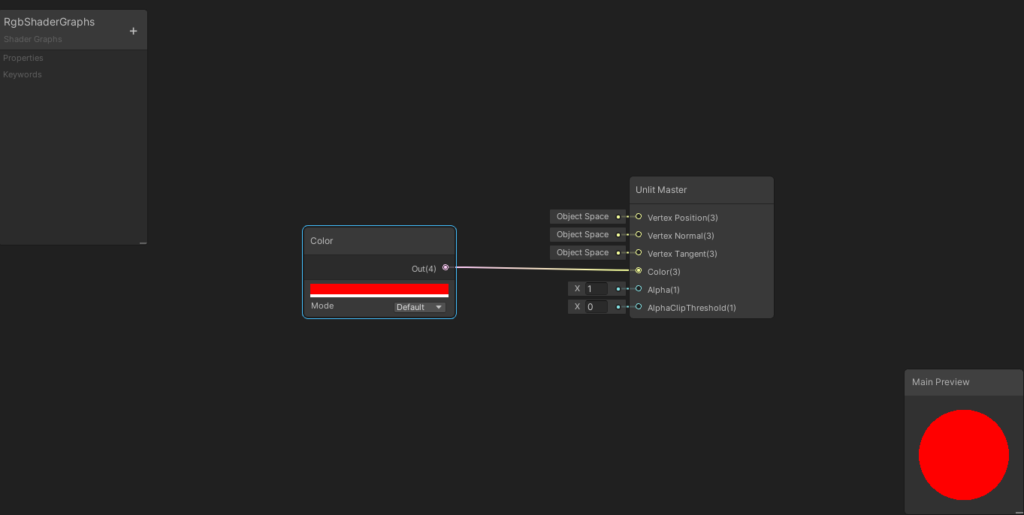使用pbr的shader graph来进行连线UE4中的基础颜色表达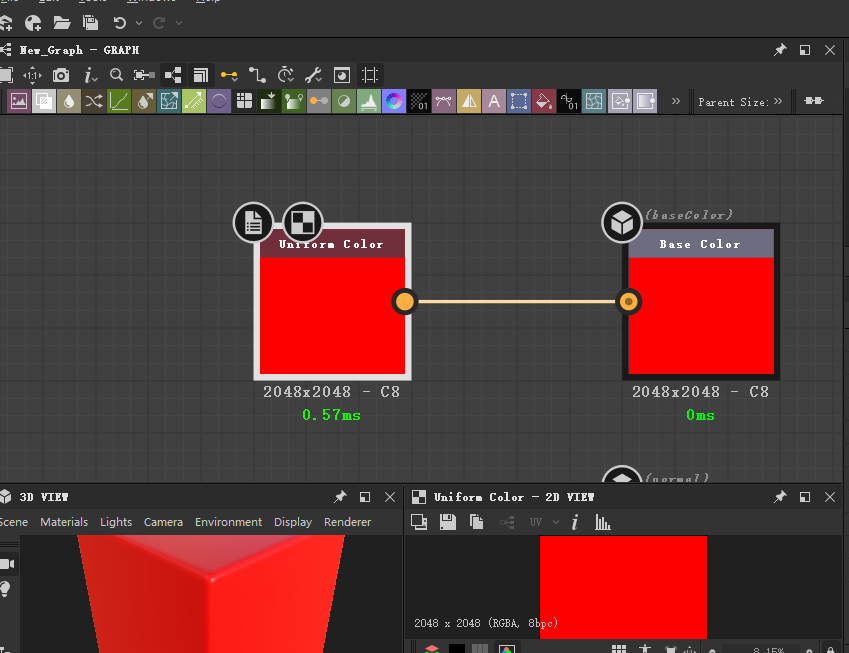SD中的基础颜色表达